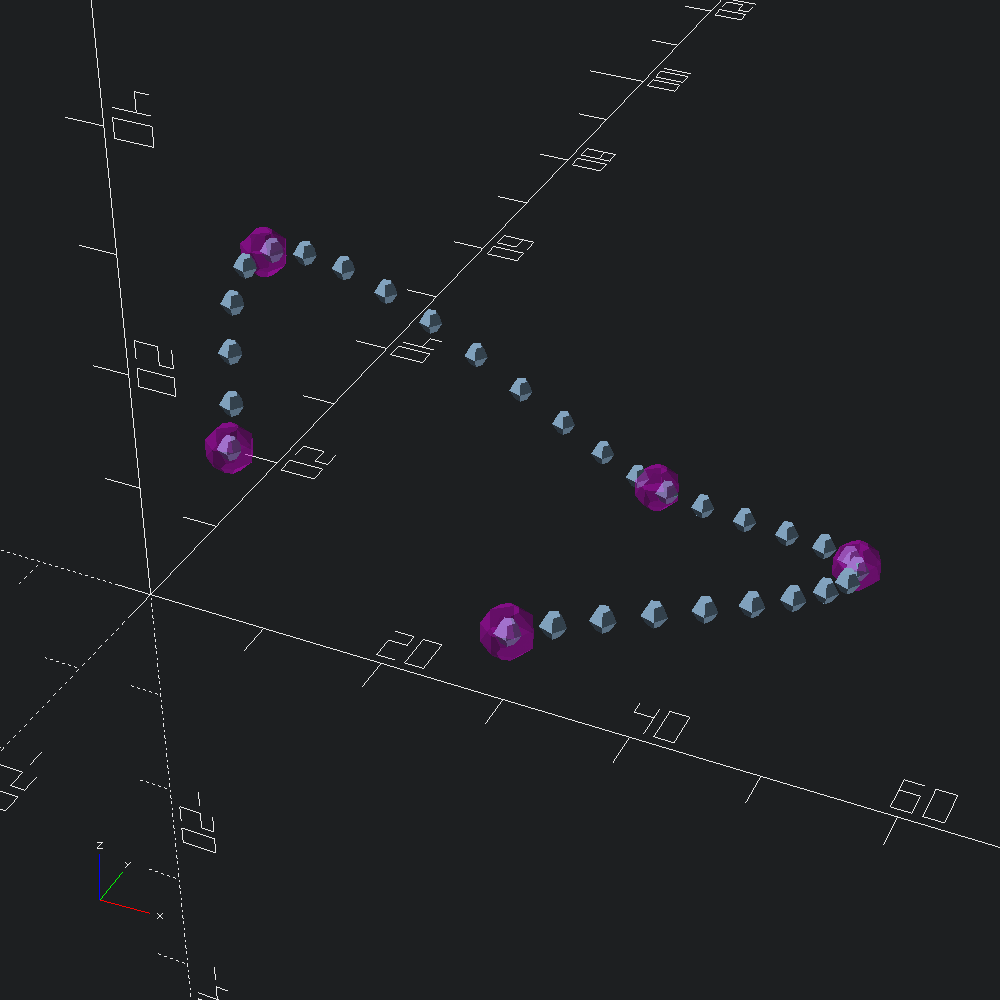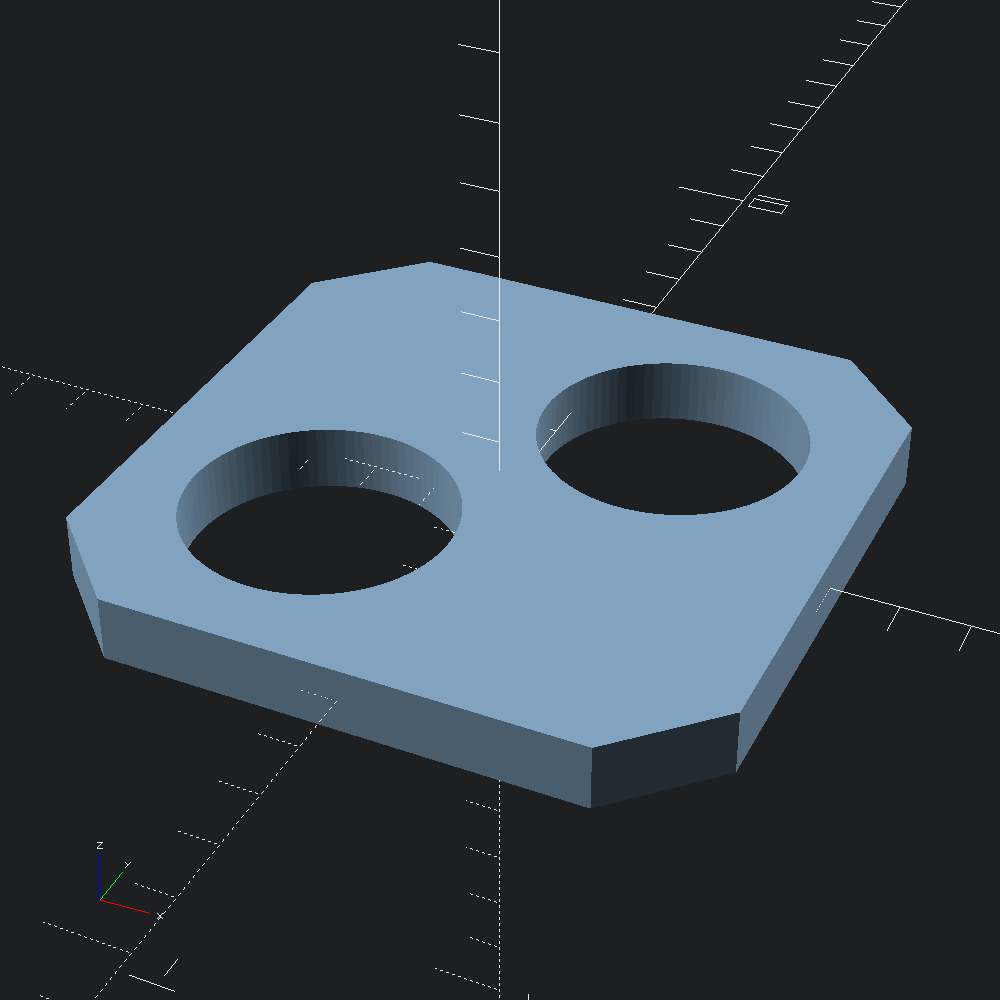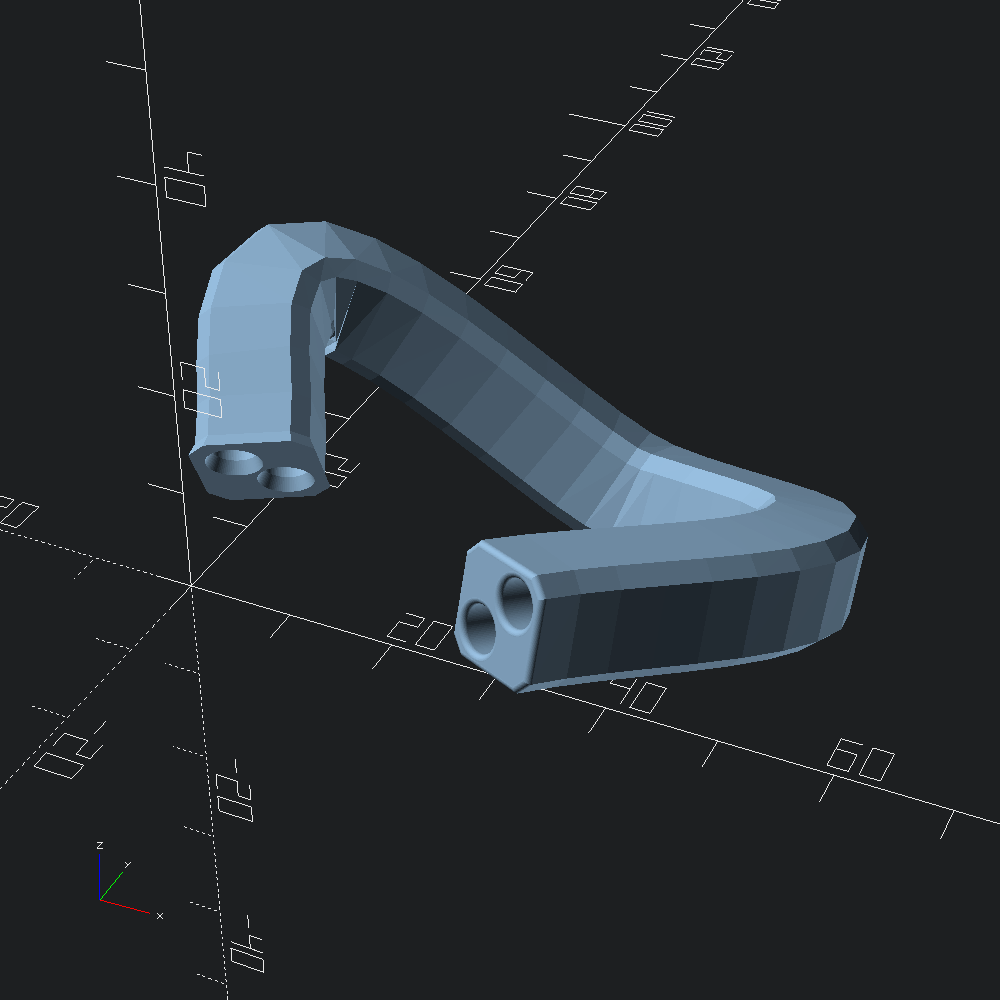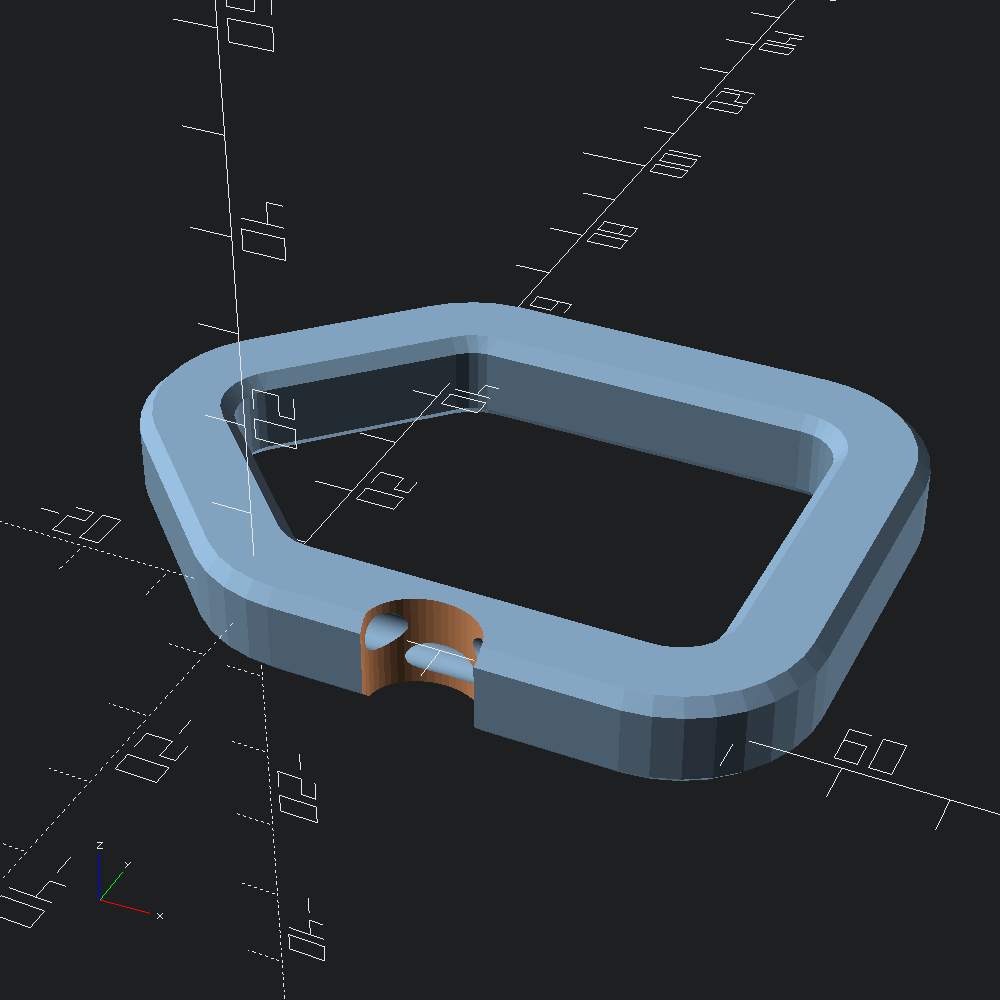# Rounded sweeps

``````open OCADml

Create a bezier spline function which passes through all of points in `control` using `Bezier3.of_path`, and interpolate `20` points along it to create our `path` with `Bezier3.curve`.

``````let control =
V3.[ v 5. 5. 12.; v 0. 20. 20.; v 30. 30. 0.; v 50. 20. 5.; v 35. (-10.) 15. ]

let path = Bezier3.curve ~fn:30 @@ Bezier3.of_path control``````

We can quickly visualize `path`, and the `control` points that it passes through by using the `Debug.show_path3` helper, which places a `Scad.d3` shape at each point along the path (this takes a function from index to shape, rather than a shape directly to allow for differentiating the points, e.g. numbering with `Scad.text`).

``````let () =
[ Debug.show_path3 (fun _ -> Scad.sphere 1.) path
; Debug.show_path3
(fun _ -> Scad.(color ~alpha:0.3 Color.Magenta @@ sphere 2.))
control
]``````Draw a 2d polygon with a chamfered square outline, and two circular holes. Chamfering the square outer path is accomplished via `Path2.roundover`, which takes a `Path2.Round.t` specifation, built here using the `Path2.Round.flat` constructor, that tells `Path2.roundover` to apply `~corner` to all of the points of the given path.

``````let poly =
let holes =
let s = Path2.circle ~fn:90 2.
and d = 1.9 in
Path2.[ translate (v2 (-.d) (-.d)) s; translate (v2 d d) s ]
and outer =
Path2.square ~center:true (v2 10. 10.)
|> Path2.Round.(flat ~corner:(chamf (`Width 2.)))
|> Path2.roundover
in
Poly2.make ~holes outer``````

2d shapes defined with `Poly2.t` can be translated into OpenSCAD polygons by way of `Poly2.to_scad`.

``let () = Scad.to_file "chamfered_square_with_holes.scad" (Scad.of_poly2 poly)```Mesh.sweep` derived functions take a `~caps` parameter that specifies what to do with the end faces of the extruded mesh. By default, both caps are flat and identical to the input polygon (`Mesh.Cap.flat_caps`), but in this example, we will be rounding them over.

To build our `caps`, we'll use the `Mesh.Cap.capped` constructor which takes specification types for how we would like to treat the bottom and top faces of our extrusion. In this case, we'll be applying roundovers to both the `bot`tom and `top` faces, so we use `Mesh.Cap.round` which takes an `Mesh.Cap.offsets` containing the offset distance/radius and "vertical" step for each outline of the outer roundover, and optionally, the desired treatment of the inner paths (as `~holes`). Typically, the offsets will be generated by a helper such as `Mesh.Cap.chamf` and `Mesh.Cap.circ` below.

Here we apply a negative (outward flaring) chamfer to the bottom face, setting `holes` to ``Same`, so that the circular holes in `poly` are also expanded, rather than pinched off (default is (`~holes:`Flip`), which negates the outer roundover for inner paths). For the top face, we specify a positive (inward) circular roundover, leaving `holes` as its default since we want the holes to flare out instead.

``````let caps =
Mesh.Cap.(
capped
~bot:(round ~holes:`Same @@ chamf ~height:(-1.2) ~angle:(Float.pi /. 8.) ())
~top:(round @@ circ (`Radius 0.5)) )``````

Extrude `poly` along `path`, with rounding over the end caps according to `caps` using `Mesh.path_extrude`.

``let mesh = Mesh.path_extrude ~path ~caps poly``

Convert our mesh into an OpenSCAD polyhedron and output to file with `Scad.of_mesh`.

``let () = Scad.to_file "rounded_polyhole_sweep.scad" (Scad.of_mesh mesh)``Rounded 3d paths for sweeping can also be drawn with the help of the `Path3.Round` module, which works much the same as its counterpart in `Path2` that we used to chamfer our `poly` earlier.

``````let rounded_path =
Path3.(
roundover ~fn:32
@@ Round.flat
~closed:true
(v3 (-25.) 25. 0. :: Path3.square (v2 50. 50.)) )``````

`Mesh.path_extrude` (and other functions derived from `Mesh.sweep`) can be given `~caps:`Looped`, which will connect the final position of the sweep up with the beginning, rather than sealing both ends off with caps. If the swept polygon has holes (as our `poly` does), they will be included, and continuous much like the outer shape.

``````let () =
let loop = Scad.of_mesh @@ Mesh.(path_extrude ~caps:Cap.looped ~path:rounded_path poly)
and cut =